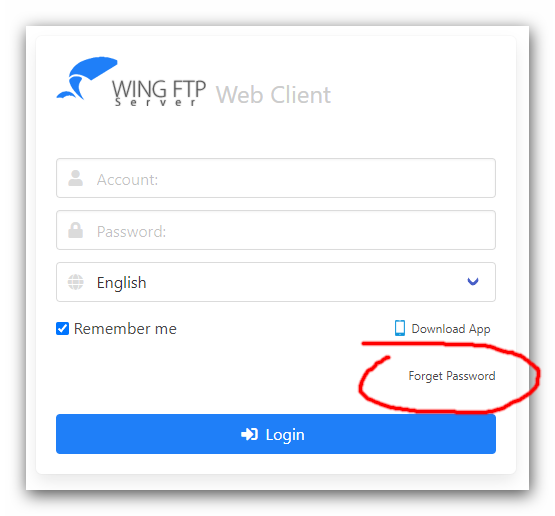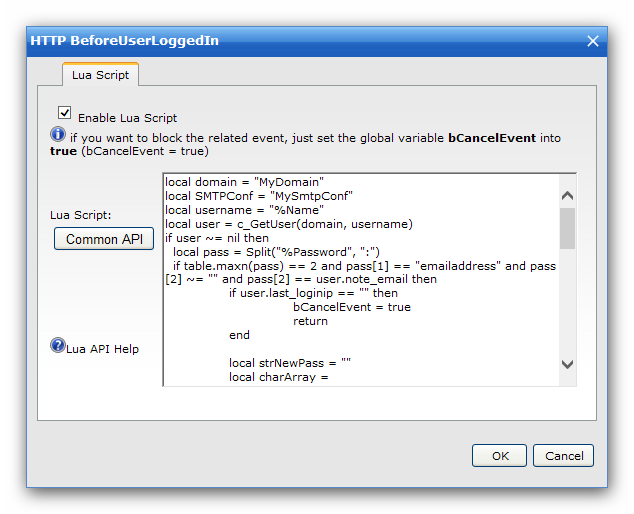Page 1 of 1

Posted: Mon May 30, 2022 7:05 am
First, replace the login page with a modified version for the web client, just download and unzip this zip file into the folder "/webclient": https://www.wftpserver.com/bbsres/resetpass.zip

Then the web client's login page will look like this:If your clients forget the login password, they can click the button "Forget Password", fill out the form and click the button "Reset Password". If the requested name and email address are correct, a new password will be sent to client's mailbox.

So the client's email address must be defined at "Domain -> Users -> Edit a user -> Notes -> Email address".

Next, you should insert the below Lua script into "Event Manager -> HTTP Events -> BeforeUserLoggedIn":

Code: Select all

``````local domain = "MyDomain"
local SMTPConf = "MySmtpConf"
if user ~= nil then
if table.maxn(pass) == 2 and pass == "emailaddress" and pass ~= "" and pass == user.note_email then
bCancelEvent = true
return
end

local strNewPass = ""
local charArray = {'0','1','2','3','4','5','6','7','8','9','a','b','c','d','e','f','g','h','i','j','k','l','m','n','o','p','q','r','s','t','u','v','w','x','y','z','~','!','@','#','\$','^','&'}
math.randomseed(c_GetRandom())
math.random()
for i=1,10 do
local nowindex = math.random(1,table.maxn(charArray))
strNewPass = strNewPass..charArray[nowindex]
end

if bMailSent == true then
for k,v in pairs(user) do
if type(v) == "boolean" then
if v == true then
user[k] = 1
else
user[k] = 0
end
end
end